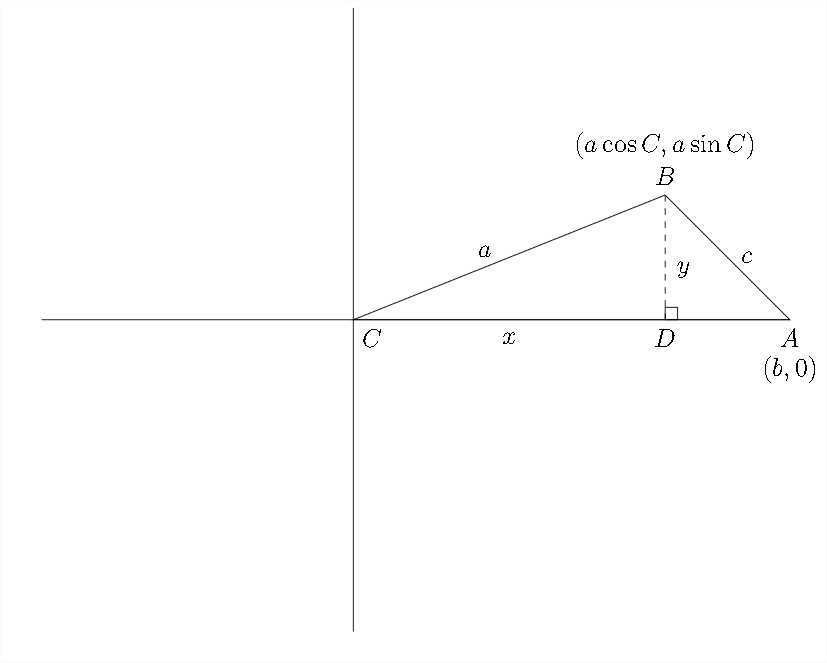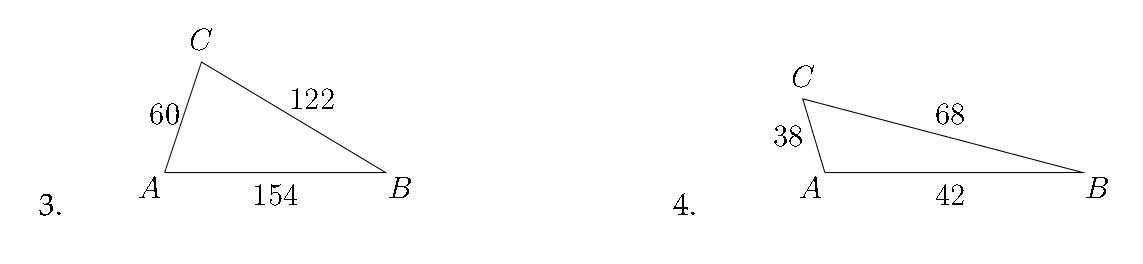# 11.3: The Law of Cosines

$$\newcommand{\vecs}{\overset { \rightharpoonup} {\mathbf{#1}} }$$ $$\newcommand{\vecd}{\overset{-\!-\!\rightharpoonup}{\vphantom{a}\smash {#1}}}$$$$\newcommand{\id}{\mathrm{id}}$$ $$\newcommand{\Span}{\mathrm{span}}$$ $$\newcommand{\kernel}{\mathrm{null}\,}$$ $$\newcommand{\range}{\mathrm{range}\,}$$ $$\newcommand{\RealPart}{\mathrm{Re}}$$ $$\newcommand{\ImaginaryPart}{\mathrm{Im}}$$ $$\newcommand{\Argument}{\mathrm{Arg}}$$ $$\newcommand{\norm}{\| #1 \|}$$ $$\newcommand{\inner}{\langle #1, #2 \rangle}$$ $$\newcommand{\Span}{\mathrm{span}}$$ $$\newcommand{\id}{\mathrm{id}}$$ $$\newcommand{\Span}{\mathrm{span}}$$ $$\newcommand{\kernel}{\mathrm{null}\,}$$ $$\newcommand{\range}{\mathrm{range}\,}$$ $$\newcommand{\RealPart}{\mathrm{Re}}$$ $$\newcommand{\ImaginaryPart}{\mathrm{Im}}$$ $$\newcommand{\Argument}{\mathrm{Arg}}$$ $$\newcommand{\norm}{\| #1 \|}$$ $$\newcommand{\inner}{\langle #1, #2 \rangle}$$ $$\newcommand{\Span}{\mathrm{span}}$$$$\newcommand{\AA}{\unicode[.8,0]{x212B}}$$

There are situations in which the Law of sines cannot be used to solve a triangle. In the diagram below, we have information about two sides and the included angle:The problem above lacks a complete angle-side pair which is necessary to set up the Law of sines calculation.

Another common situation involves a triangle in which all three sides are known but no angles are known:Again, the lack of an angle-side pair would prevent us from setting up a Law of sines calculation.

The Law of Cosines is one way to get around this difficulty. Using the Law of cosines is more complicated than using the Law of sines, however, as we have just seen, the Law of sines will not always be enough to solve a triangle.

To derive The Law of cosines, we begin with an arbitrary triangle, like the one seen on the next page:In this diagram we have taken the arbitrary triangle and created a perpendicular with length $$y .$$ From this, we can say that $$\sin C=\frac{y}{a}$$ and that $$a \sin C=y$$

We can split side $$A C$$ into two pieces $$A D$$ and $$C D,$$ as seen below, and label the distance $$C D$$ as $$x$$Then, we can say that $$\cos C=\frac{x}{a}$$ and that $$a \cos C=x$$
If we then put this triangle onto the coordinate axes with $$\angle C$$ at the origin (0,0) we can derive the Law of cosines. The coordinate of the vertex at $$\angle B$$ will be $$(a \cos C, a \sin C),$$ and the coordinates of the vertex at $$\angle A$$ will be $$(b, 0)$$Using the distance formula, we can say that:
$c=\sqrt{(a \cos C-b)^{2}+(a \sin C-0)^{2}}$ Squaring both sides:
$c^{2}=(a \cos C-b)^{2}+(a \sin C-0)^{2}$ and
$c^{2}=a^{2} \cos ^{2} C-2 a b \cos C+b^{2}+a^{2} \sin ^{2} C$ or
$c^{2}=a^{2} \sin ^{2} C+a^{2} \cos ^{2} C+b^{2}-2 a b \cos C$

Factoring out the $$a^{2}$$ and replacing $$\sin ^{2} C+\cos ^{2} C$$ with 1 , we come out with one of the most common forms of the Law of cosines:
$\begin{array}{c} c^{2}=a^{2} \sin ^{2} C+a^{2} \cos ^{2} C+b^{2}-2 a b \cos C \\ c^{2}=a^{2}\left(\sin ^{2} C+\cos ^{2} C\right)+b^{2}-2 a b \cos C \\ c^{2}=a^{2}(1)+b^{2}-2 a b \cos C \\ c^{2}=a^{2}+b^{2}-2 a b \cos C \end{array}$ Any letter may be used to represent each of the sides, but the order that the letters are used in is very important. The side of the triangle isolated on the left hand side must correspond to the angle used on the right hand side.

The Law of cosines
$\begin{array}{l} a^{2}=b^{2}+c^{2}-2 b c \cos A \\ b^{2}=a^{2}+c^{2}-2 a c \cos B \\ c^{2}=a^{2}+b^{2}-2 a b \cos C \end{array}$

We'll look at three examples- two in which two sides and the included angle are given and one in which the three sides of the triangle are given.

Example 1
Solve the triangle: $$\angle A=38^{\circ}, c=17, b=8$$ Round angle measures and side lengths to the nearest $$10^{\text {th }}$$.It's usually a good idea to see if you can use the Law of sines first, since it is easier to calculate. In this case we can't because we don't have a complete angle-side pair. So, using the Law of cosines to find side $$a:$$
$\begin{array}{c} a^{2}=b^{2}+c^{2}-2 b c \cos A \\ a^{2}=8^{2}+17^{2}-2 * 8 * 17 * \cos 38^{\circ} \\ a^{2} \approx 64+289-272 * 0.7880 \\ a^{2} \approx 353-214.336 \\ a^{2} \approx 138.664 \\ a \approx 11.8 \end{array}$

Once we know that $$a \approx 11.8$$ we can use this to find the other angles using the Law of sines. Because of the issue of the ambiguous case in using the Law of sines, it's often a good idea to find the angles that correspond to the two shortest sides in the triangle, because if there is an obtuse angle in the triangle it will have to correspond to the longest side. If we find the two smaller angles, we can be assured that they will both be acute and we can subtract from $$180^{\circ}$$ to find the largest angle.

$\begin{array}{c} \frac{\sin 38^{\circ}}{11.8}=\frac{\sin B}{8} \\ 8 \times \frac{0.61566}{11.8}=\sin B \\ 0.4174 \approx \sin B \\ 24.7^{\circ} \approx B \end{array}$ So with $$\angle A=38^{\circ}$$ and $$\angle B \approx 24.7^{\circ},$$ then:
$\angle C \approx 180^{\circ}-\left(38^{\circ}+24.7^{\circ}\right) \approx 180^{\circ}-62.7^{\circ} \approx 117.3^{\circ}$

So, the angles and sides of the triangle would be:
$\begin{array}{lll} \angle A=38^{\circ} & a \approx 11.8 \\ \angle B \approx 24.7^{\circ} & b=8 \\ \angle C \approx 117.3^{\circ} & c=17 \end{array}$ In example $$2,$$ we'll look at a problem in which an obtuse angle is given.

Example 2
Solve the triangle: $$\angle A=110^{\circ}, c=30, b=35$$ Round angle measures and side lengths to the nearest $$10^{\text {th }}$$.The calculation for this problem is slightly different from the last one because the cosine of $$110^{\circ}$$ will be negative:
$\begin{array}{c} a^{2}=b^{2}+c^{2}-2 b c \cos A \\ a^{2}=35^{2}+30^{2}-2 * 35 * 30 * \cos 110^{\circ} \\ a^{2} \approx 1225+900-2100 *(-0.3420) \\ a^{2} \approx 2125+718.2 \\ a^{2} \approx 2843.2 \\ a \approx 53.3 \end{array}$

In this problem, since we were given an obtuse angle, then the other two angles must be acute and we don't have to worry about the ambiguous case in using the Law of sines.

$\frac{\sin 110^{\circ}}{53.3}=\frac{\sin B}{35} \quad \frac{\sin 110^{\circ}}{53.3}=\frac{\sin C}{30}$

$35 * \frac{0.9397}{53.3}=\sin B \quad 30 * \frac{0.9397}{53.3}=\sin C$

$0.61706 \approx \sin B \quad 0.5289 \approx \sin C$

$38.1^{\circ} \approx B \quad 31.9^{\circ} \approx C$

So the angles and sides of the triangle would be:
$\begin{array}{lll} \angle A=110^{\circ} & a \approx 53.3 \\ \angle B \approx 38.1^{\circ} & b=35 \\ \angle C \approx 31.9^{\circ} & c=30 \end{array}$

In example $$3,$$ we'll look at a problem in which three side lengths are given and we find an angle using the Law of cosines.
Example 3
Solve the triangle: $$a=20, \quad c=9, \quad b=15$$ Round angle measures to the nearest $$10^{t h}$$It doesn't matter which angle we choose to solve, but whichever angle we choose must correspond to the side isolated on the left-hand side of the formula. If we want to solve for $$\angle B,$$ we would say:
$\begin{array}{c} b^{2}=a^{2}+c^{2}-2 a c \cos B \\ 15^{2}=20^{2}+9^{2}-2 * 20 * 9 * \cos B \\ 225=400+81-360 * \cos B \\ 225=481-360 \cos B \\ -256=-360 \cos B \\ \frac{-256}{-360}=\frac{-360 \cos B}{-360} \\ 0.7 \overline{1}=\cos B \\ 44.7^{\circ} \approx B \end{array}$ Once we know the measure of $$\angle B,$$ we'll use this to find the measure of $$\angle C,$$ which corresponds to side $$c,$$ the smallest side. Then we'll subtract to find the biggest angle.
\begin{aligned} \frac{\sin 44.7^{\circ}}{15} &=\frac{\sin C}{9} \\ 9 * \frac{0.7034}{15} &=\sin C \\ 0.42204 &\approx \sin C & \\ 25.0^{\circ} & \approx C \end{aligned}

So, with $$\angle B \approx 44.7^{\circ}$$ and $$\angle C \approx 25.0^{\circ},$$ then:
$\angle A \approx 180^{\circ}-\left(44.7^{\circ}+25.0^{\circ}\right) \approx 180^{\circ}-69.7^{\circ} \approx 110.3^{\circ}$ So the angles and sides of the triangle would be:
$\begin{array}{lll} \angle A=110.3^{\circ} & a \approx 53.3 \\ \angle B \approx 44.7^{\circ} & b=35 \\ \angle C \approx 25.0^{\circ} & c=30 \end{array}$

If we had used the Law of sines to find $$\angle A$$, the calculator would have returned the value of the reference angle for $$\angle A$$, rather than the angle that is actually in the triangle described in the problem!

Exercises 4.3
In each problem, solve the triangle. Round side lengths to the nearest $$100^{\text {th }}$$ and angle measures to the nearest $$10^{\text {th }}$$.5. $$\quad \angle A=52^{\circ}, \quad c=27, \quad b=36$$
6. $$\quad \angle B=75^{\circ}, \quad a=32, \quad c=59$$
7. $$\quad \angle B=135^{\circ}, \quad a=12, \quad c=18$$
8. $$\quad \angle C=120^{\circ}, \quad b=22, \quad a=30$$
9. $$\quad a=21, \quad b=26, \quad c=23$$
10. $$\quad a=11, \quad b=13, \quad c=17$$
11. $$\quad a=25, \quad b=32, \quad c=40$$
12. $$\quad a=60, \quad b=88, \quad c=120$$
13. $$\quad \angle A=77.4^{\circ}, \quad b=444, \quad c=390$$
14. $$\quad \angle B=10^{\circ}, \quad a=18, \quad c=30$$
15. $$\quad a=112.7, \quad b=96.5, \quad c=130.2$$
16. $$\quad a=4.7, \quad b=3.2, \quad c=5.9$$

This page titled 11.3: The Law of Cosines is shared under a CC BY-NC-SA 4.0 license and was authored, remixed, and/or curated by Richard W. Beveridge.